# Implementation of Quick sort using MPI, OMP and Posix thread

• Last Updated : 01 Nov, 2021

QuickSort is a Divide and Conquer Algorithm. It picks an element as a pivot and partitions the array around the picked pivot. There are many ways of choosing the pivot elements. They are:

• Always pick the first element as a pivot.
• Always pick the last element as the pivot (implemented below)
• Pick a random element as a pivot.
• Pick median as a pivot.

MPI: MPI stands for Message Passing Interface. Here the message is data. MPI allows data to be passed between processes in a distributed memory environment. In C, “mpi.h” is a header file that includes all data structures, routines, and constants of MPI. Using “mpi.h” parallelized the quick sort algorithm.  Below is the C program to implement quicksort using MPI:

## C

 `// C program to implement the Quick Sort` `// Algorithm using MPI` `#include ` `#include ` `#include ` `#include ` `#include ` `using` `namespace` `std;`   `// Function to swap two numbers` `void` `swap(``int``* arr, ``int` `i, ``int` `j)` `{` `    ``int` `t = arr[i];` `    ``arr[i] = arr[j];` `    ``arr[j] = t;` `}`   `// Function that performs the Quick Sort` `// for an array arr[] starting from the` `// index start and ending at index end` `void` `quicksort(``int``* arr, ``int` `start, ``int` `end)` `{` `    ``int` `pivot, index;`   `    ``// Base Case` `    ``if` `(end <= 1)` `        ``return``;`   `    ``// Pick pivot and swap with first` `    ``// element Pivot is middle element` `    ``pivot = arr[start + end / 2];` `    ``swap(arr, start, start + end / 2);`   `    ``// Partitioning Steps` `    ``index = start;`   `    ``// Iterate over the range [start, end]` `    ``for` `(``int` `i = start + 1; i < start + end; i++) {`   `        ``// Swap if the element is less` `        ``// than the pivot element` `        ``if` `(arr[i] < pivot) {` `            ``index++;` `            ``swap(arr, i, index);` `        ``}` `    ``}`   `    ``// Swap the pivot into place` `    ``swap(arr, start, index);`   `    ``// Recursive Call for sorting` `    ``// of quick sort function` `    ``quicksort(arr, start, index - start);` `    ``quicksort(arr, index + 1, start + end - index - 1);` `}`   `// Function that merges the two arrays` `int``* merge(``int``* arr1, ``int` `n1, ``int``* arr2, ``int` `n2)` `{` `    ``int``* result = (``int``*)``malloc``((n1 + n2) * ``sizeof``(``int``));` `    ``int` `i = 0;` `    ``int` `j = 0;` `    ``int` `k;`   `    ``for` `(k = 0; k < n1 + n2; k++) {` `        ``if` `(i >= n1) {` `            ``result[k] = arr2[j];` `            ``j++;` `        ``}` `        ``else` `if` `(j >= n2) {` `            ``result[k] = arr1[i];` `            ``i++;` `        ``}`   `        ``// Indices in bounds as i < n1` `        ``// && j < n2` `        ``else` `if` `(arr1[i] < arr2[j]) {` `            ``result[k] = arr1[i];` `            ``i++;` `        ``}`   `        ``// v2[j] <= v1[i]` `        ``else` `{` `            ``result[k] = arr2[j];` `            ``j++;` `        ``}` `    ``}` `    ``return` `result;` `}`   `// Driver Code` `int` `main(``int` `argc, ``char``* argv[])` `{` `    ``int` `number_of_elements;` `    ``int``* data = NULL;` `    ``int` `chunk_size, own_chunk_size;` `    ``int``* chunk;` `    ``FILE``* file = NULL;` `    ``double` `time_taken;` `    ``MPI_Status status;`   `    ``if` `(argc != 3) {` `        ``printf``(``"Desired number of arguments are not their "` `               ``"in argv....\n"``);` `        ``printf``(``"2 files required first one input and "` `               ``"second one output....\n"``);` `        ``exit``(-1);` `    ``}`   `    ``int` `number_of_process, rank_of_process;` `    ``int` `rc = MPI_Init(&argc, &argv);`   `    ``if` `(rc != MPI_SUCCESS) {` `        ``printf``(``"Error in creating MPI "` `               ``"program.\n "` `               ``"Terminating......\n"``);` `        ``MPI_Abort(MPI_COMM_WORLD, rc);` `    ``}`   `    ``MPI_Comm_size(MPI_COMM_WORLD, &number_of_process);` `    ``MPI_Comm_rank(MPI_COMM_WORLD, &rank_of_process);`   `    ``if` `(rank_of_process == 0) {` `        ``// Opening the file` `        ``file = ``fopen``(argv, ``"r"``);`   `        ``// Printing Error message if any` `        ``if` `(file == NULL) {` `            ``printf``(``"Error in opening file\n"``);` `            ``exit``(-1);` `        ``}`   `        ``// Reading number of Elements in file ...` `        ``// First Value in file is number of Elements` `        ``printf``(` `            ``"Reading number of Elements From file ....\n"``);` `        ``fscanf``(file, ``"%d"``, &number_of_elements);` `        ``printf``(``"Number of Elements in the file is %d \n"``,` `               ``number_of_elements);`   `        ``// Computing chunk size` `    ``chunk_size = (number_of_elements % ` `                  ``number_of_process == 0) ? ` `                  ``(number_of_elements / ` `                   ``number_of_process) : ` `                  ``(number_of_elements /` `                  ``(number_of_process - 1);`   `    ``data = (``int` `*)``malloc``(number_of_process * ` `                         ``chunk_size * ` `                         ``sizeof``(``int``));` `    `  `    ``// Reading the rest elements in which ` `    ``// operation is being performed` `    ``printf``(``"Reading the array from the file.......\n"``);` `    ``for``(``int` `i = 0; i < number_of_elements; i++)` `    ``{` `            ``fscanf``(file, ``"%d"``, &data[i]);` `    ``}`   `    ``// Padding data with zero` `    ``for``(``int` `i = number_of_elements;` `            ``i < number_of_process * ` `                ``chunk_size; i++)` `    ``{` `            ``data[i] = 0;` `    ``}`   `    ``// Printing the array read from file` `      ``printf``(``"Elements in the array is : \n"``);` `      ``for``(``int` `i = 0; i < number_of_elements; i++)` `      ``{` `            ``printf``(``"%d  "``, data[i]);` `      ``}`   `      ``printf``(``"\n"``);`   `      ``fclose``(file);` `      ``file = NULL;` `    ``}`   `    ``// Blocks all process until reach this point` `    ``MPI_Barrier(MPI_COMM_WORLD);`   `    ``// Starts Timer` `    ``time_taken -= MPI_Wtime();`   `    ``// BroadCast the Size to all the` `    ``// process from root process` `    ``MPI_Bcast(&number_of_elements, 1, MPI_INT, 0,` `              ``MPI_COMM_WORLD);`   `    ``// Computing chunk size` `  ``chunk_size= (number_of_elements % ` `               ``number_of_process == 0) ? ` `              ``(number_of_elements / ` `               ``number_of_process) : ` `              ``(number_of_elements / ` `              ``(number_of_process - 1);`   `  ``// Calculating total size of chunk ` `  ``// according to bits` `  ``chunk = (``int` `*)``malloc``(chunk_size * ` `                        ``sizeof``(``int``));`   `  ``// Scatter the chuck size data to all process` `  ``MPI_Scatter(data, chunk_size, MPI_INT, chunk, ` `              ``chunk_size, MPI_INT, 0, MPI_COMM_WORLD);` `  ``free``(data);` `  ``data = NULL;`   `  ``// Compute size of own chunk and` `               ``// then sort them ` `  ``// using quick sort`   `  ``own_chunk_size = (number_of_elements >= ` `                    ``chunk_size*(rank_of_process + 1)) ? ` `                    ``chunk_size : (number_of_elements - ` `                                  ``chunk_size*rank_of_process);`   `  ``// Sorting array with quick sort for every ` `  ``// chunk as called by process` `  ``quicksort(chunk, 0, own_chunk_size);`   `  ``for``(``int` `step = 1; step < number_of_process; step = 2 * step)` `  ``{` `        ``if` `(rank_of_process % (2 * step) != 0) {` `            ``MPI_Send(chunk, own_chunk_size, MPI_INT,` `                     ``rank_of_process - step, 0,` `                     ``MPI_COMM_WORLD);` `            ``break``;` `        ``}`   `        ``if` `(rank_of_process + step < number_of_process) {` `            ``int` `received_chunk_size` `                ``= (number_of_elements` `                   ``>= chunk_size` `                          ``* (rank_of_process + 2 * step))` `                      ``? (chunk_size * step)` `                      ``: (number_of_elements` `                         ``- chunk_size` `                               ``* (rank_of_process + step));` `            ``int``* chunk_received;` `            ``chunk_received = (``int``*)``malloc``(` `                ``received_chunk_size * ``sizeof``(``int``));` `            ``MPI_Recv(chunk_received, received_chunk_size,` `                     ``MPI_INT, rank_of_process + step, 0,` `                     ``MPI_COMM_WORLD, &status);`   `            ``data = merge(chunk, own_chunk_size,` `                         ``chunk_received,` `                         ``received_chunk_size);`   `            ``free``(chunk);` `            ``free``(chunk_received);` `            ``chunk = data;` `            ``own_chunk_size` `                ``= own_chunk_size + received_chunk_size;` `        ``}` `  ``}`   `  ``// Stop the timer` `  ``time_taken += MPI_Wtime();`   `  ``// Opening the other file as taken form input ` `  ``// and writing it to the file and giving it ` `  ``// as the output` `  ``if``(rank_of_process == 0)` `  ``{` `        ``// Opening the file` `        ``file = ``fopen``(argv, ``"w"``);`   `        ``if` `(file == NULL) {` `            ``printf``(``"Error in opening file... \n"``);` `            ``exit``(-1);` `        ``}`   `        ``// Printing total number of elements` `        ``// in the file` `        ``fprintf``(` `            ``file,` `            ``"Total number of Elements in the array : %d\n"``,` `            ``own_chunk_size);`   `        ``// Printing the value of array in the file` `        ``for` `(``int` `i = 0; i < own_chunk_size; i++) {` `            ``fprintf``(file, ``"%d  "``, chunk[i]);` `        ``}`   `        ``// Closing the file` `        ``fclose``(file);`   `        ``printf``(``"\n\n\n\nResult printed in output.txt file "` `               ``"and shown below: \n"``);`   `        ``// For Printing in the terminal` `        ``printf``(``"Total number of Elements given as input : "` `               ``"%d\n"``,` `               ``number_of_elements);` `        ``printf``(``"Sorted array is: \n"``);`   `        ``for` `(``int` `i = 0; i < number_of_elements; i++) {` `            ``printf``(``"%d  "``, chunk[i]);` `        ``}`   `        ``printf``(` `            ``"\n\nQuicksort %d ints on %d procs: %f secs\n"``,` `            ``number_of_elements, number_of_process,` `            ``time_taken);` `  ``}`   `  ``MPI_Finalize();` `  ``return` `0;` `}`

Output: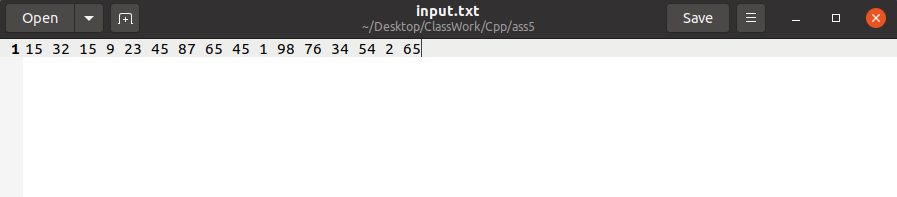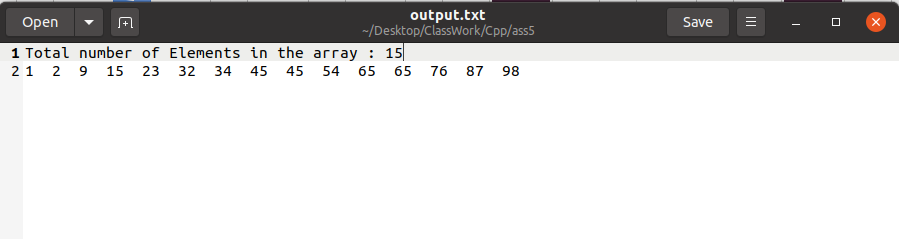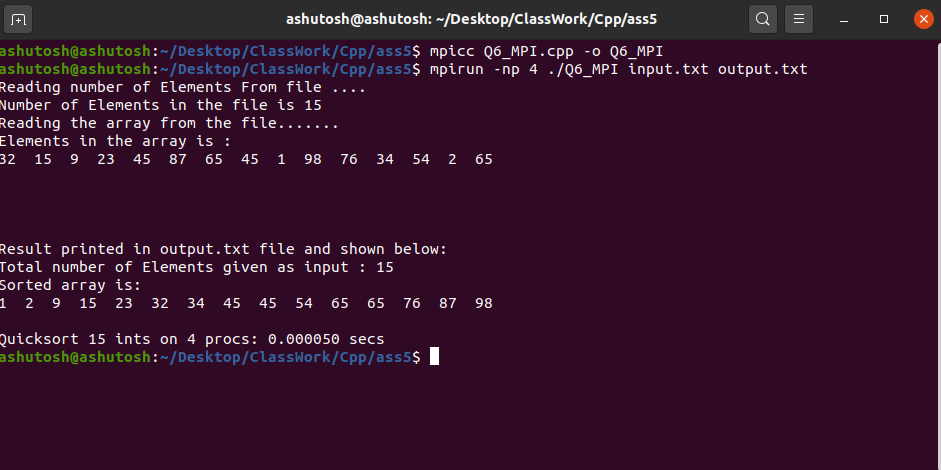OMP: OMP is Open Multi-Processing. It’s an Application Program Interface (API) that may be used to explicitly direct multi-threaded, shared memory parallelism. In C/ C++, “omp.h” is a header file that includes all the related directives related to OMP. Using “omp.h” parallelized quick sort. Below is the C++ program to implement the above concept:

## C++

 `// C++ program to implement the Quick Sort` `// using OMI` `#include ` `#include ` `using` `namespace` `std;`   `// Function to swap two numbers a and b` `void` `swap(``int``* a, ``int``* b)` `{` `    ``int` `t = *a;` `    ``*a = *b;` `    ``*b = t;` `}`   `// Function to perform the partitioning` `// of array arr[]` `int` `partition(``int` `arr[], ``int` `start, ``int` `end)` `{` `    ``// Declaration` `    ``int` `pivot = arr[end];` `    ``int` `i = (start - 1);`   `    ``// Rearranging the array` `    ``for` `(``int` `j = start; j <= end - 1; j++) {` `        ``if` `(arr[j] < pivot) {` `            ``i++;` `            ``swap(&arr[i], &arr[j]);` `        ``}` `    ``}` `    ``swap(&arr[i + 1], &arr[end]);`   `    ``// Returning the respective index` `    ``return` `(i + 1);` `}`   `// Function to perform QuickSort Algorithm` `// using openmp` `void` `quicksort(``int` `arr[], ``int` `start, ``int` `end)` `{` `    ``// Declaration` `    ``int` `index;`   `    ``if` `(start < end) {`   `        ``// Getting the index of pivot` `        ``// by partitioning` `        ``index = partition(arr, start, end);`   `// Parallel sections` `#pragma omp parallel sections` `        ``{` `#pragma omp section` `            ``{` `                ``// Evaluating the left half` `                ``quicksort(arr, start, index - 1);` `            ``}` `#pragma omp section` `            ``{` `                ``// Evaluating the right half` `                ``quicksort(arr, index + 1, end);` `            ``}` `        ``}` `    ``}` `}`   `// Driver Code` `int` `main()` `{` `    ``// Declaration` `    ``int` `N;`   `    ``// Taking input the number of` `    ``// elements we wants` `    ``cout << ``"Enter the number of elements"` `         ``<< ``" you want to Enter\n"``;` `    ``cin >> N;`   `    ``// Declaration of array` `    ``int` `arr[N];`   `    ``cout << ``"Enter the array: \n"``;`   `    ``// Taking input that array` `    ``for` `(``int` `i = 0; i < N; i++) {` `        ``cin >> arr[i];` `    ``}`   `    ``// Calling quicksort having parallel` `    ``// code implementation` `    ``quicksort(arr, 0, N - 1);`   `    ``// Printing the sorted array` `    ``cout << ``"Array after Sorting is: \n"``;`   `    ``for` `(``int` `i = 0; i < N; i++) {` `        ``cout << arr[i] << ``" "``;` `    ``}`   `    ``return` `0;` `}`

Output: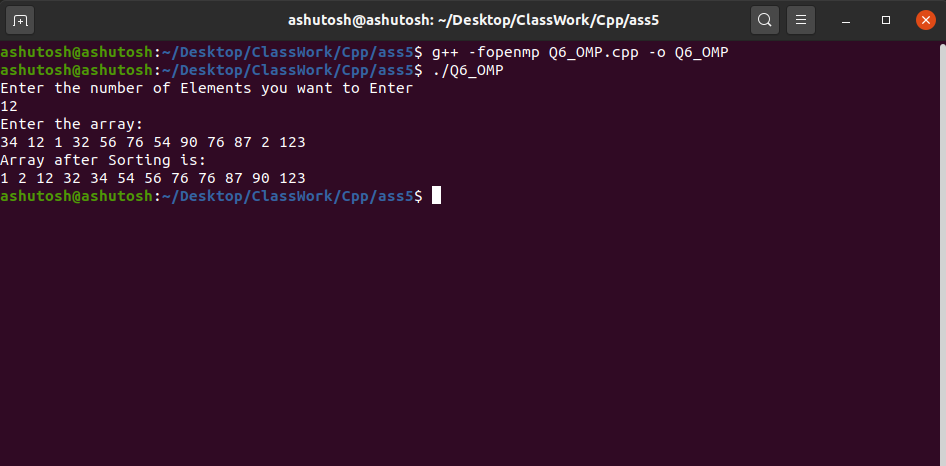POSIX Threads: The POSIX thread libraries are a C/C++ thread API based on standards. It enables the creation of a new concurrent process flow. It works well on multi-processor or multi-core systems, where the process flow may be scheduled to execute on another processor, increasing speed through parallel or distributed processing. Below is the C++ program to implement quicksort using POSIX threads:

## C++14

 `// C++ program to implement the Quick Sort` `// using POSIX Thread` `#include ` `#include ` `using` `namespace` `std;`   `// Structure` `struct` `data_set {` `    ``int` `start_index;` `    ``int` `end_index;` `    ``int``* data;` `};`   `// Function to perform swap operations` `void` `swap(``int``* a, ``int``* b)` `{` `    ``int` `t = *a;` `    ``*a = *b;` `    ``*b = t;` `}`   `// Partition function for making` `// partition in array` `int` `partition(``int` `arr[], ``int` `left_index,` `              ``int` `right_index)` `{` `    ``// Declaration and initialization` `    ``// choosing pivot element form which` `    ``// we make partition`   `    ``// Here pivot is last element of` `    ``// the array` `    ``int` `pivot = arr[right_index];` `    ``int` `i = left_index - 1;`   `    ``// Making array as per requirement` `    ``// arranging element smaller than` `    ``// pivot on left side and larger` `    ``// then pivot on right side` `    ``for` `(``int` `j = left_index;` `         ``j <= right_index - 1; j++) {`   `        ``if` `(arr[j] < pivot) {` `            ``i++;` `            ``swap(&arr[i], &arr[j]);` `        ``}` `    ``}`   `    ``swap(&arr[i + 1], &arr[right_index]);`   `    ``// Returning the partition index` `    ``return` `i + 1;` `}`   `// Quicksort Function for sorting` `// array` `void``* quick_sort(``void``* data)` `{` `    ``// Retrieving back the data sent` `    ``// from thread` `    ``struct` `data_set* info = (``struct` `data_set*)data;`   `    ``// Declaration of left index` `    ``int` `left_index, right_index, index;`   `    ``// Initialization of left and` `    ``// right index` `    ``left_index = info->start_index;` `    ``right_index = info->end_index;`   `    ``// Recursive call of quick_sort` `    ``// function` `    ``if` `(left_index < right_index) {`   `        ``// Declaration of pthread and` `        ``// pthread attribute type object` `        ``pthread_attr_t attr;` `        ``pthread_t first_thread;` `        ``pthread_t second_thread;`   `        ``// Making two pointers of type` `        ``// data_set for making again` `        ``// call form thread` `        ``struct` `data_set* info1 = ``new` `data_set;` `        ``struct` `data_set* info2 = ``new` `data_set;`   `        ``// Their initialization` `        ``info1->data = info->data;` `        ``info2->data = info->data;`   `        ``// Initialize of pthread attribute` `        ``pthread_attr_init(&attr);`   `        ``// For setting the set detach` `        ``// state of attribute` `        ``pthread_attr_setdetachstate(` `            ``&attr, PTHREAD_CREATE_JOINABLE);`   `        ``// Partition the array for any` `        ``// recursive call` `        ``index = partition(info->data,` `                          ``left_index,` `                          ``right_index);`   `        ``info1->start_index = left_index;` `        ``info1->end_index = index - 1;`   `        ``// Create pthread type object and` `        ``// printing the error if any` `        ``if` `(pthread_create(&first_thread,` `                           ``&attr, quick_sort,` `                           ``info1)) {` `            ``cout << ``"Error in creating thread "` `                 ``<< endl;`   `            ``// Exiting in case of not` `            ``// creation of thread` `            ``exit``(-1);` `        ``}`   `        ``info2->start_index = index + 1;` `        ``info2->end_index = right_index;`   `        ``// Creating pthread type object` `        ``// and print the error` `        ``if` `(pthread_create(&second_thread,` `                           ``&attr, quick_sort,` `                           ``info2)) {` `            ``cout << ``"Error in creating thread "` `                 ``<< endl;`   `            ``// Exiting in case of not` `            ``// creation of thread` `            ``exit``(-1);` `        ``}`   `        ``// Joining the threads` `        ``pthread_join(first_thread, NULL);` `        ``pthread_join(second_thread, NULL);` `    ``}`   `    ``return` `NULL;` `}`   `// Driver Code` `int` `main()` `{` `    ``// Declaration of Number of threads` `    ``int` `N;`   `    ``struct` `data_set* info = ``new` `data_set;`   `    ``// Taking number of elements as input` `    ``cout << ``"Enter number of elements"` `         ``<< ``" in the array: \n"``;` `    ``cin >> N;`   `    ``// Declaration of array` `    ``int` `A[N];`   `    ``// Initialization of array` `    ``cout << ``"Enter the array: "` `<< endl;` `    ``for` `(``int` `i = 0; i < N; i++) {` `        ``cin >> A[i];` `    ``}`   `    ``// Initialize of structure of` `    ``// data_set type` `    ``info->data = A;` `    ``info->start_index = 0;` `    ``info->end_index = N - 1;`   `    ``// Declaration of pthread object` `    ``pthread_t thread_id;`   `    ``// Creating and pthread object and` `    ``// printing the array of any` `    ``if` `(pthread_create(&thread_id, NULL,` `                       ``quick_sort,` `                       ``info)) {` `        ``cout << ``"Error in creating thread"` `             ``<< endl;`   `        ``// Exit in case of error` `        ``exit``(-1);` `    ``}`   `    ``// Joining the pthread object` `    ``int` `r1 = pthread_join(thread_id, NULL);`   `    ``// Printing the array if any in case` `    ``// of joining` `    ``if` `(r1) {` `        ``cout << ``"Error in Joinging thread"` `             ``<< endl;`   `        ``// Exiting in case of error` `        ``exit``(-1);` `    ``}`   `    ``// Printing the array after sorting` `    ``cout << ``"Sorted Array is: "` `<< endl;`   `    ``for` `(``int` `i = 0; i < N; i++) {` `        ``cout << A[i] << ``" "``;` `    ``}` `    ``cout << endl;`   `    ``// Exiting from pthread programming` `    ``pthread_exit(NULL);`   `    ``return` `0;` `}`

Output: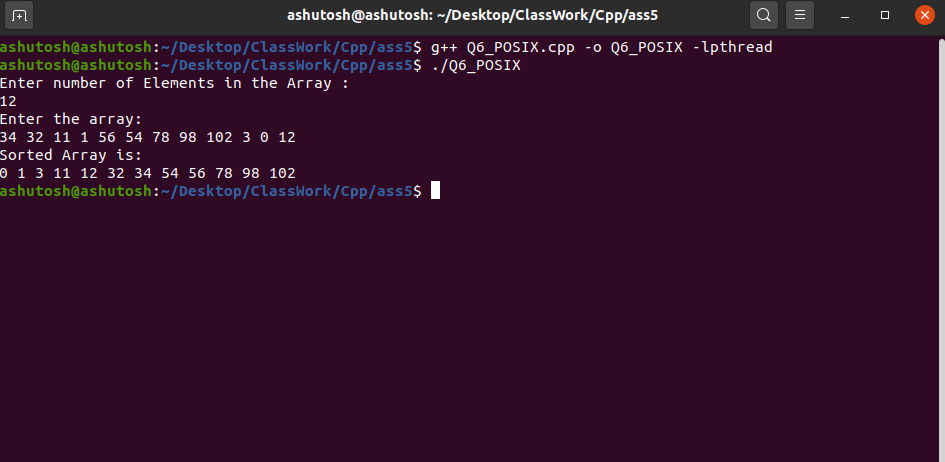My Personal Notes arrow_drop_up
Recommended Articles
Page :